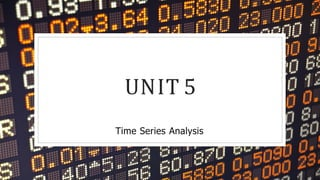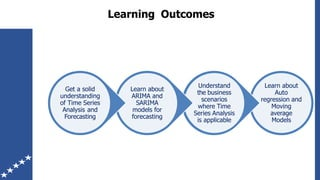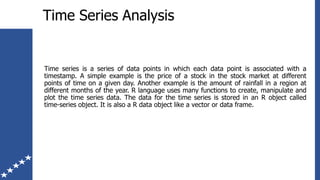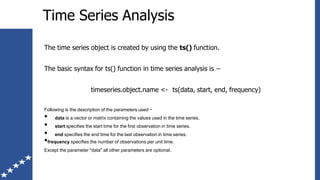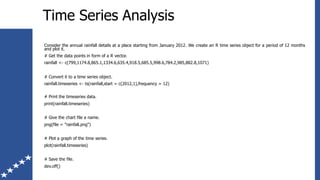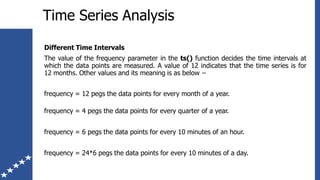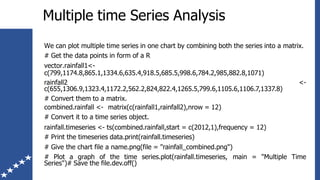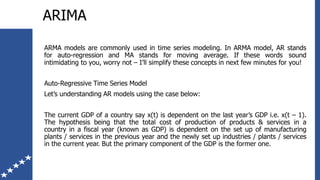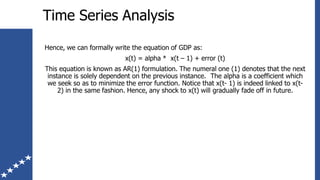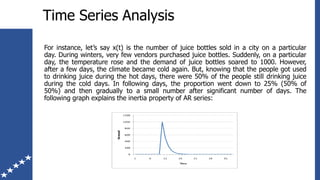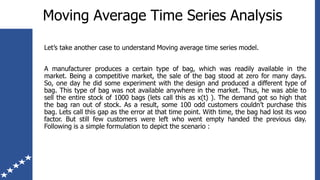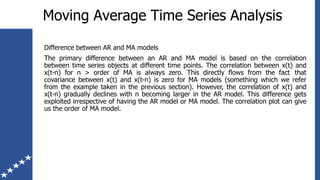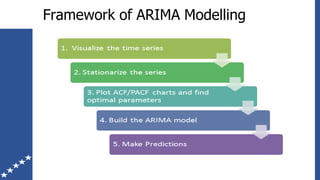1 of 13

### Unit5_Time Series Analysis.pdf

1. UNIT 5 Time Series Analysis
2. Learn about Auto regression and Moving average Models Understand the business scenarios where Time Series Analysis is applicable Learn about ARIMA and SARIMA models for forecasting Get a solid understanding of Time Series Analysis and Forecasting Learning Outcomes
3. Time Series Analysis Time series is a series of data points in which each data point is associated with a timestamp. A simple example is the price of a stock in the stock market at different points of time on a given day. Another example is the amount of rainfall in a region at different months of the year. R language uses many functions to create, manipulate and plot the time series data. The data for the time series is stored in an R object called time-series object. It is also a R data object like a vector or data frame.
4. Time Series Analysis The time series object is created by using the ts() function. The basic syntax for ts() function in time series analysis is − timeseries.object.name <- ts(data, start, end, frequency) Following is the description of the parameters used − • data is a vector or matrix containing the values used in the time series. • start specifies the start time for the first observation in time series. • end specifies the end time for the last observation in time series. •frequency specifies the number of observations per unit time. Except the parameter "data" all other parameters are optional.
5. Time Series Analysis Consider the annual rainfall details at a place starting from January 2012. We create an R time series object for a period of 12 months and plot it. # Get the data points in form of a R vector. rainfall <- c(799,1174.8,865.1,1334.6,635.4,918.5,685.5,998.6,784.2,985,882.8,1071) # Convert it to a time series object. rainfall.timeseries <- ts(rainfall,start = c(2012,1),frequency = 12) # Print the timeseries data. print(rainfall.timeseries) # Give the chart file a name. png(file = "rainfall.png") # Plot a graph of the time series. plot(rainfall.timeseries) # Save the file. dev.off()
6. Time Series Analysis Different Time Intervals The value of the frequency parameter in the ts() function decides the time intervals at which the data points are measured. A value of 12 indicates that the time series is for 12 months. Other values and its meaning is as below − frequency = 12 pegs the data points for every month of a year. frequency = 4 pegs the data points for every quarter of a year. frequency = 6 pegs the data points for every 10 minutes of an hour. frequency = 24*6 pegs the data points for every 10 minutes of a day.
7. Multiple time Series Analysis We can plot multiple time series in one chart by combining both the series into a matrix. # Get the data points in form of a R vector.rainfall1<- c(799,1174.8,865.1,1334.6,635.4,918.5,685.5,998.6,784.2,985,882.8,1071) <- rainfall2 c(655,1306.9,1323.4,1172.2,562.2,824,822.4,1265.5,799.6,1105.6,1106.7,1337.8) # Convert them to a matrix. combined.rainfall <- matrix(c(rainfall1,rainfall2),nrow = 12) # Convert it to a time series object. rainfall.timeseries <- ts(combined.rainfall,start = c(2012,1),frequency = 12) # Print the timeseries data.print(rainfall.timeseries) # Give the chart file a name.png(file = "rainfall_combined.png") # Plot a graph of the time series.plot(rainfall.timeseries, main = "Multiple Time Series")# Save the file.dev.off()
8. ARIMA ARMA models are commonly used in time series modeling. In ARMA model, AR stands for auto-regression and MA stands for moving average. If these words sound intimidating to you, worry not – I’ll simplify these concepts in next few minutes for you! Auto-Regressive Time Series Model Let’s understanding AR models using the case below: The current GDP of a country say x(t) is dependent on the last year’s GDP i.e. x(t – 1). The hypothesis being that the total cost of production of products & services in a country in a fiscal year (known as GDP) is dependent on the set up of manufacturing plants / services in the previous year and the newly set up industries / plants / services in the current year. But the primary component of the GDP is the former one.
9. Time Series Analysis Hence, we can formally write the equation of GDP as: x(t) = alpha * x(t – 1) + error (t) This equation is known as AR(1) formulation. The numeral one (1) denotes that the next instance is solely dependent on the previous instance. The alpha is a coefficient which we seek so as to minimize the error function. Notice that x(t- 1) is indeed linked to x(t- 2) in the same fashion. Hence, any shock to x(t) will gradually fade off in future.
10. Time Series Analysis For instance, let’s say x(t) is the number of juice bottles sold in a city on a particular day. During winters, very few vendors purchased juice bottles. Suddenly, on a particular day, the temperature rose and the demand of juice bottles soared to 1000. However, after a few days, the climate became cold again. But, knowing that the people got used to drinking juice during the hot days, there were 50% of the people still drinking juice during the cold days. In following days, the proportion went down to 25% (50% of 50%) and then gradually to a small number after significant number of days. The following graph explains the inertia property of AR series:
11. Moving Average Time Series Analysis Let’s take another case to understand Moving average time series model. A manufacturer produces a certain type of bag, which was readily available in the market. Being a competitive market, the sale of the bag stood at zero for many days. So, one day he did some experiment with the design and produced a different type of bag. This type of bag was not available anywhere in the market. Thus, he was able to sell the entire stock of 1000 bags (lets call this as x(t) ). The demand got so high that the bag ran out of stock. As a result, some 100 odd customers couldn’t purchase this bag. Lets call this gap as the error at that time point. With time, the bag had lost its woo factor. But still few customers were left who went empty handed the previous day. Following is a simple formulation to depict the scenario :
12. Moving Average Time Series Analysis Difference between AR and MA models The primary difference between an AR and MA model is based on the correlation between time series objects at different time points. The correlation between x(t) and x(t-n) for n > order of MA is always zero. This directly flows from the fact that covariance between x(t) and x(t-n) is zero for MA models (something which we refer from the example taken in the previous section). However, the correlation of x(t) and x(t-n) gradually declines with n becoming larger in the AR model. This difference gets exploited irrespective of having the AR model or MA model. The correlation plot can give us the order of MA model.
13. Framework of ARIMA Modelling